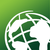# Multiple line return of multiple VB if then statements

1180
5
04-19-2017 07:33 AMOccasional Contributor

I've been tasked with creating labels for some monitoring wells, the labels are the well name and then a list of a years worth of sulfate concentrations in each well, this is easy enough to do, however they want the text to be red if the sulfate value is above a certain concentration. I wrote a vbscript block with multiple if then statements to try to achieve this, and while it shows no errors, it only returns the results of the last if/then statement. What am I missing? I imagine it may need some kind of instruction to return each individual statement?

Function FindLabel ([Well_Nme], [Total_Sulfate_3_16], [Total_Sulfate_6_16], [Total_Sulfate_9_16], [Total_Sulfate_12_16])
FindLabel="<BOL><UND>"&[Well_Nme]&"</UND></BOL>"
if ( [Total_Sulfate_3_16]=>250000) then
FindLabel= "<CLR red='255'>"& [Total_Sulfate_3_16] &"</CLR>"
elseif ([Total_Sulfate_3_16] <250000) then
Findlabel = [Total_Sulfate_3_16]
end if
if ([Total_Sulfate_6_16] =>250000) then
FindLabel = "<CLR red='255'>"& [Total_Sulfate_6_16] &"</CLR>"
elseif ( [Total_Sulfate_6_16] <250000) then
Findlabel = [Total_Sulfate_6_16]
end if
if ( [Total_Sulfate_9_16] =>250000) then
FindLabel = "<CLR red='255'>"& [Total_Sulfate_9_16] &"</CLR>"
elseif ( [Total_Sulfate_9_16] <250000) then
Findlabel = [Total_Sulfate_9_16]
end if
if ( [Total_Sulfate_12_16]=>250000) then
FindLabel = "<CLR red='255'>"& [Total_Sulfate_12_16] &"</CLR>"
elseif ( [Total_Sulfate_12_16]<250000) then
Findlabel = [Total_Sulfate_12_16]
end if
End Function

Tags (2)
5 RepliesbyMVP Regular Contributor

Rather than stand-alone If statments, use the If...elseif...elseif format structure.

Regards,

JimOccasional Contributor III

What is your program that you use? ArcGIS desktop,Pro , ..?Occasional Contributor

DesktopbyMVP Regular Contributor

After a second look, I know that arcMap typically uses ">=" instead of "=>", and the "=>" will generate problems with query expression. Perhaps that is preventing you from getting the results you expect?by
Occasional Contributor III
``````Function FindLabel ([Well_Nme], [Total_Sulfate_3_16], [Total_Sulfate_6_16], [Total_Sulfate_9_16], [Total_Sulfate_12_16])
FindLabel="<BOL><UND>"&[Well_Nme]&"</UND></BOL>" & vbcr
if ( [Total_Sulfate_3_16]>=250000) then
FindLabel= FindLabel & "<CLR red='255'>"& [Total_Sulfate_3_16] &"</CLR>" & vbcr
else
FindLabel = Findlabel & [Total_Sulfate_3_16] & vbcr
end if
if ([Total_Sulfate_6_16] >=250000) then
FindLabel = FindLabel & "<CLR red='255'>"& [Total_Sulfate_6_16] &"</CLR>" & vbcr
else
FindLabel = FindLabel & [Total_Sulfate_6_16] & vbcr
end if
if ( [Total_Sulfate_9_16] >=250000) then
FindLabel = Findlabel & "<CLR red='255'>"& [Total_Sulfate_9_16] &"</CLR>" & vbcr
else
FindLabel = Findlabel & [Total_Sulfate_9_16] & vbcr
end if
if ( [Total_Sulfate_12_16]>=250000) then
FindLabel = FindLabel & "<CLR red='255'>"& [Total_Sulfate_12_16] &"</CLR>"
else
FindLabel = FindLabel & [Total_Sulfate_12_16]
end if
End Function‍‍‍‍‍‍‍‍‍‍‍‍‍‍‍‍‍‍‍‍‍‍‍‍‍‍‍‍‍‍‍‍‍‍‍‍‍‍‍‍‍‍‍‍‍‍‍‍‍‍‍‍‍‍‍‍‍‍‍‍‍‍‍‍‍‍‍‍‍‍‍‍‍‍‍‍‍‍‍‍‍‍‍‍‍‍‍‍‍‍‍‍‍‍‍‍‍‍‍‍‍‍‍‍‍‍‍‍‍‍‍‍‍‍‍‍‍‍‍‍‍‍‍‍‍‍‍‍‍‍‍‍‍‍‍‍‍‍``````

In your code every time you set FindLabel you are erasing the previous values....  you only needed to set FindLabel = Findlabel & "whatever else you wanted to add on"